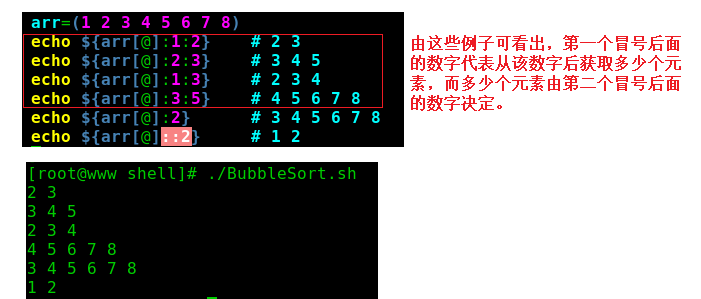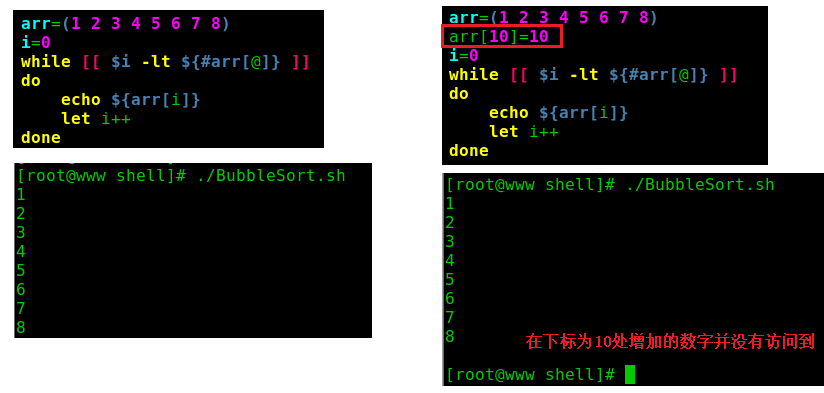# shell脚本中数组用法总结及脚本实现冒泡排序

1.shell脚本中数组的操作

（1）shell脚本中数组的定义

arr=(1 2 3 4 5 6 7 )

（2）默认获取数组中第一个元素

echo $arr 或者 echo${arr}

（3）通过下标访问取出数组中的元素

echo ${arr} //shell数组下标是从0开始，所以这里取的是数组中第三个元素 （4）访问整个数组元素 echo${arr[@]}    or    echo ${arr[*]} （5）获取数组的长度 echo${#arr[@]}

（6）获取单个元素字符串长度

echo ${#arr} （7）切片方式获取一部分数组内容 echo${arr[@]:1:2}

（8）从第二个元素开始

echo ${arr[@]:2} （9）到第三个元素 echo${arr[@]::2}2.总结一下在使用shell脚本中数组时要注意的地方

（1）只支持一维数组，下标从0开始；

（2）在shell中，用圆括号来表示数组，数组元素用“空格”符号分隔开；

（3）可以不使用连续的下标，而且下标的范围没有限制；（例如：a=(1 2 3 4 ) 在下标为9位置处再插入一个数a=10）

（4）${#arr[@]}可得出数组的长度，但是在数组不连续的时候用数组长度去遍历易出错。3.脚本实现冒泡排序 #!/bin/bash echo "Please input a number list" read -a arr for((i=0;i<${#arr[@]};++i))
do
for((j=${#arr[@]}-1;j>i;--j)) do if [[${arr[j]} -lt ${arr[j-1]} ]] then tmp=${arr[j]};
arr[j]=${arr[j-1]}; arr[j-1]=$tmp;
fi
done
done

echo "after sorting!"
echo ${arr[@]}  也可这样写： #!/bin/bash echo "Please input a number list!" read -a arr for((i=0;i<${#arr[@]};++i))
do
for((j=i;j<${#arr[@]}-i-1;++j)) do if [[${arr[j]} -gt ${arr[j+1]} ]] then tmp=${arr[j]}
arr[j]=${arr[j+1]} arr[j+1]=$tmp
fi
done
done
echo "after sorting!"
echo \${arr[@]}